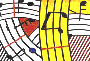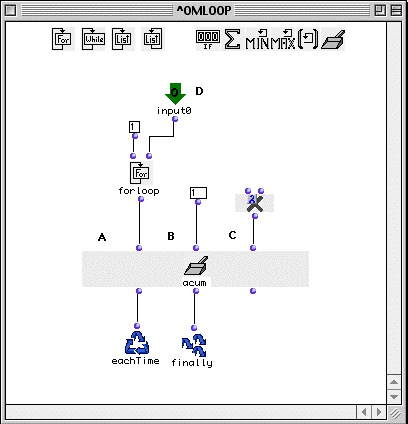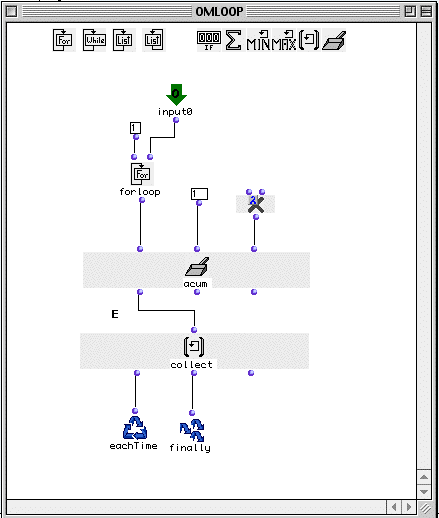Tutorial 36 - Omloop III

Accumulation

Topics

omloop using the acum collector

Functions used

omloop and om*Description

We will examine in this tutorial a simple accumulative computation. We will take as an example the factorial arithmetical operation. The factorial operartion on a number is:

5! (five factorial) = 1*2*3*4*5 =120

the operation consists in multiplying an initial integer (1) by an arithmetical serie. For example, multiplying 1*1 then the result by 2, then the result by 3, etc... In order to do this, we will need a special iteration allowing to hold the result in a 'buffer' and compute it whenever we have an iteration. This is possible in the omloop control function while using acum.

Patch structureA: The acum collector takes three arguments, the first being a list that will control iteration. Here we will construct an arithmetical series starting by 1 up till our (D) input, which in this example is 5. In order to do that we will use forloop which returns at each step an integer between <from> and <to> included. When the value <to> is reached and returned, iteration stops.

B: In B acum asks for an initial argument that will be the starting value of its buffer

C: As its third argument acum asks for a binary function. (A function with only two arguments and not less). We will use in our case the om* function in lambda state.

 Step listloop output Value of thefirst input of accumulator Value of the second input of accumulator Internal state (buffer) Binary function "om*" output 0 1 1 1 1 1 1 2 2 / 1 2 2 3 3 / 2 6 3 4 4 / 6 24 4 5 5 / 24 120 120

Inner state of acum

The result of the operation is the resulting value of the internal state of acum's buffer. This is returned by the omloop usinf finally. In the case we want to have each internal state of the buffer, we must modify our omloop by adding collect as shown below.Here collect (E) is connected to the first output of accum in order to launch each iteration. Then collect is in its turn connected to eachtime and to finally. this will return the internal state of acum's buffer for each step of iteration:

OM->(1 2 6 24 120)

(For further examples of acum please consult the OMReference guide).